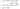# Bubble Sort Algorithm

May 16, 2019This is kind of preliminary technique of sorting. And, this is the first algorithm that a beginner learns.

In this algorithm, we iterate over the array, and compare two consecutive numbers. And, if first is larger, then swap it. And, we keep on doing this till end. So, the Bubble here is the biggest element which we keep on swapping.

## Bubble Sort Algorithm

• We start with two loops. Outer loop just goes from 0 to last-1 index.
• Inner loop always starts frmo 0-index, and goes till n-1 each time.
• On first iteration, we got the biggest element at the end of the array.
• Next iteration goes till n-1 elements. Since, we already sorted the largest element at the end.
• We keep on pushing the largets element from remaining n-1 array.

See the code here:

``````public void sort(int[] arr) {
int l = arr.length;
for (int i=0; i<l-1; i++) {
for (int j=0; j<(l-i-1); j++) {
if (arr[j] > arr[j+1]) {
//swap
int t = arr[j];
arr[j] = arr[j+1];
arr[j+1] = t;
}
}
}
}``````

## Graphical Example## Key Points

• Its an in-place sorting algorithm
• Performance is usually worse than Insertion sort
• Applicable for small set of input only
• Its very simple algorithm

## Runtime complexity

The algorithm runs on O(n^2) in worst/average case.